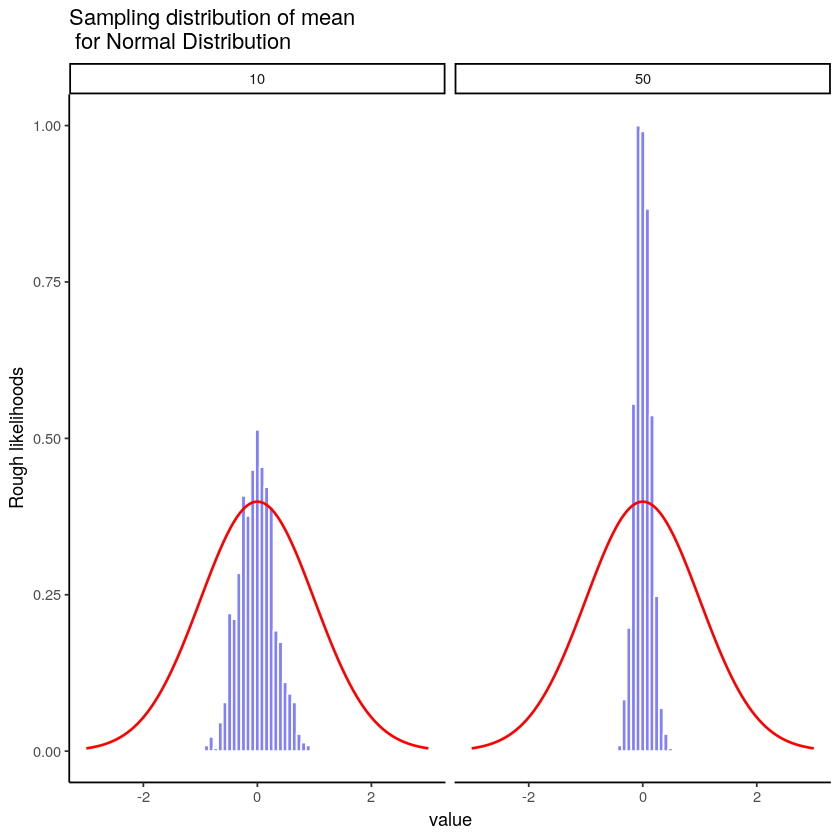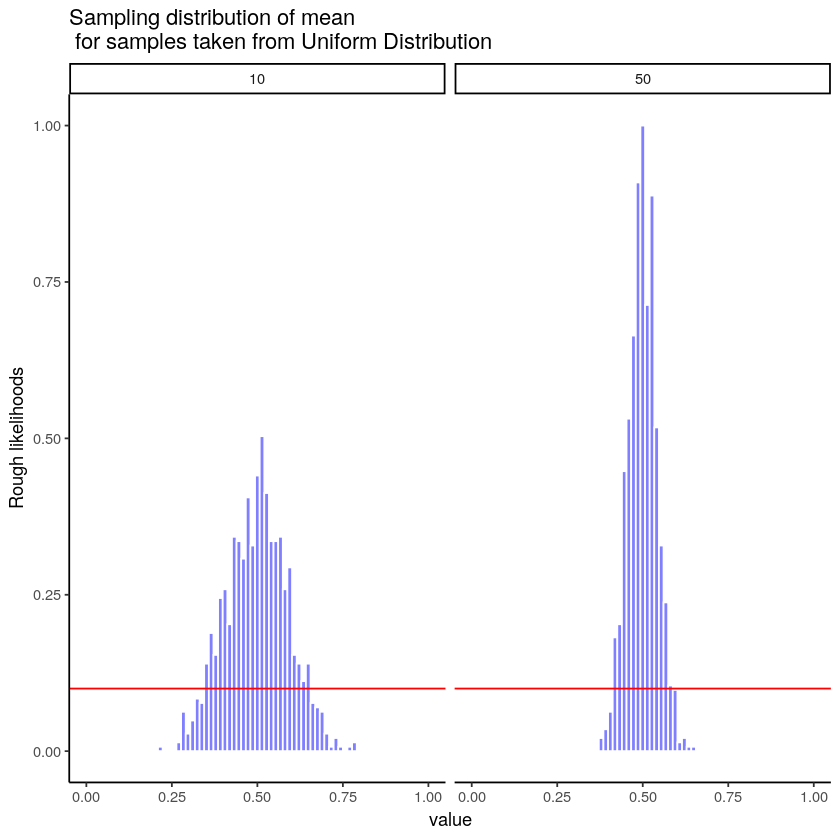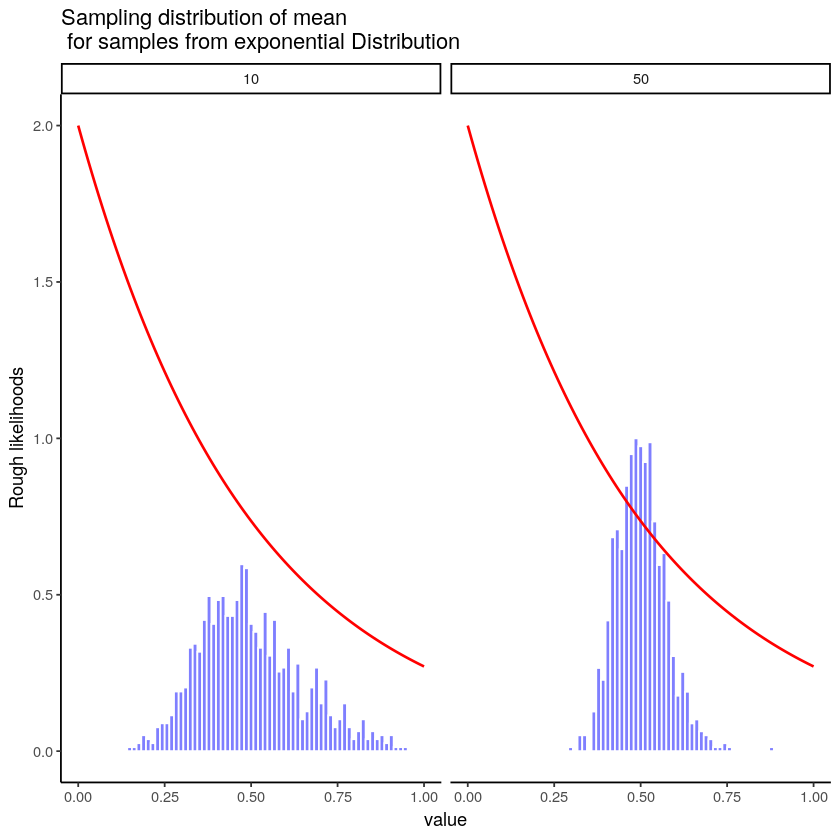# 4.11: The Central Limit Theorem

$$\newcommand{\vecs}{\overset { \rightharpoonup} {\mathbf{#1}} }$$ $$\newcommand{\vecd}{\overset{-\!-\!\rightharpoonup}{\vphantom{a}\smash {#1}}}$$$$\newcommand{\id}{\mathrm{id}}$$ $$\newcommand{\Span}{\mathrm{span}}$$ $$\newcommand{\kernel}{\mathrm{null}\,}$$ $$\newcommand{\range}{\mathrm{range}\,}$$ $$\newcommand{\RealPart}{\mathrm{Re}}$$ $$\newcommand{\ImaginaryPart}{\mathrm{Im}}$$ $$\newcommand{\Argument}{\mathrm{Arg}}$$ $$\newcommand{\norm}{\| #1 \|}$$ $$\newcommand{\inner}{\langle #1, #2 \rangle}$$ $$\newcommand{\Span}{\mathrm{span}}$$ $$\newcommand{\id}{\mathrm{id}}$$ $$\newcommand{\Span}{\mathrm{span}}$$ $$\newcommand{\kernel}{\mathrm{null}\,}$$ $$\newcommand{\range}{\mathrm{range}\,}$$ $$\newcommand{\RealPart}{\mathrm{Re}}$$ $$\newcommand{\ImaginaryPart}{\mathrm{Im}}$$ $$\newcommand{\Argument}{\mathrm{Arg}}$$ $$\newcommand{\norm}{\| #1 \|}$$ $$\newcommand{\inner}{\langle #1, #2 \rangle}$$ $$\newcommand{\Span}{\mathrm{span}}$$$$\newcommand{\AA}{\unicode[.8,0]{x212B}}$$

OK, so now you’ve seen lots of sampling distributions, and you know what the sampling distribution of the mean is. Here, we’ll focus on how the sampling distribution of the mean changes as a function of sample size.

Intuitively, you already know part of the answer: if you only have a few observations, the sample mean is likely to be quite inaccurate (you’ve already seen it bounce around): if you replicate a small experiment and recalculate the mean you’ll get a very different answer. In other words, the sampling distribution is quite wide. If you replicate a large experiment and recalculate the sample mean you’ll probably get the same answer you got last time, so the sampling distribution will be very narrow.

Let’s give ourselves a nice movie to see everything in action. We’re going to sample numbers from a normal distribution. You will see four panels, each panel represents a different sample size (n), including sample-sizes of 10, 50, 100, and 1000. The red line shows the shape of the normal distribution. The grey bars show a histogram of each of the samples that we take. The red line shows the mean of an individual sample (the middle of the grey bars). As you can see, the red line moves around a lot, especially when the sample size is small (10).

The new bits are the blue bars and the blue lines. The blue bars represent the sampling distribution of the sample mean. For example, in the panel for sample-size 10, we see a bunch of blue bars. This is a histogram of 10 sample means, taken from 10 samples of size 10. In the 50 panel, we see a histogram of 50 sample means, taken from 50 samples of size 50, and so on. The blue line in each panel is the mean of the sample means (“aaagh, it’s a mean of means”, yes it is).Figure $$\PageIndex{1}$$: Animation of samples (grey histogram shows frequency counts of data in each sample), and the sampling distribution of the mean (histogram of the sampling means for many samples). Each sample is taken from the normal distribution shown in red. The moving red line is the mean of an individual sample. The blue line is the mean of the blue histogram, which represents the sampling distribution of the mean for many samples.

What should you notice? Notice that the range of the blue bars shrinks as sample size increases. The sampling distribution of the mean is quite wide when the sample-size is 10, it narrows as sample-size increases to 50 and 100, and it’s just one bar, right in the middle when sample-size goes to 1000. What we are seeing is that the mean of the sampling distribution approaches the mean of the population as sample-size increases.

So, the sampling distribution of the mean is another distribution, and it has some variance. It varies more when sample-size is small, and varies less when sample-size is large. We can quantify this effect by calculating the standard deviation of the sampling distribution, which is referred to as the standard error. The standard error of a statistic is often denoted SE, and since we’re usually interested in the standard error of the sample mean, we often use the acronym SEM. As you can see just by looking at the movie, as the sample size $$N$$ increases, the SEM decreases.

Okay, so that’s one part of the story. However, there’s something we’ve been glossing over a little bit. We’ve seen it already, but it’s worth looking at it one more time. Here’s the thing: no matter what shape your population distribution is, as $$N$$ increases the sampling distribution of the mean starts to look more like a normal distribution. This is the central limit theorem.

To see the central limit theorem in action, we are going to look at some histograms of sample means different kinds of distributions. It is very important to recognize that you are looking at distributions of sample means, not distributions of individual samples! Here we go, starting with sampling from a normal distribution. The red line is the distribution, the blue bars are the histogram for the sample means. They both look normal!Figure $$\PageIndex{2}$$: Comparison of two normal distributions, and histograms for the sampling distribution of the mean for different samples-sizes. The range of sampling distribution of the mean shrinks as sample-size increases.

Let’s do it again. This time we sample from a flat uniform distribution. Again, we see that the distribution of the sample means is not flat, it looks like a normal distribution.Figure $$\PageIndex{3}$$: Illustration that the shape of the sampling distribution of the mean is normal, even when the samples come from a non-normal (uniform in this case) distribution.

One more time with an exponential distribution. Even though way more of the numbers should be smaller than bigger, then sampling distribution of the mean again does not look the red line. Instead, it looks more normal-ish. That’s the central limit theorem. It just works like that.Figure $$\PageIndex{4}$$: Illustration that the shape of the sampling distribution of the mean is normal, even when the samples come from a non-normal (exponential in this case) distribution.

On the basis of these figures, it seems like we have evidence for all of the following claims about the sampling distribution of the mean:

• The mean of the sampling distribution is the same as the mean of the population
• The standard deviation of the sampling distribution (i.e., the standard error) gets smaller as the sample size increases
• The shape of the sampling distribution becomes normal as the sample size increases

As it happens, not only are all of these statements true, there is a very famous theorem in statistics that proves all three of them, known as the central limit theorem. Among other things, the central limit theorem tells us that if the population distribution has mean $$\mu$$ and standard deviation $$\sigma$$, then the sampling distribution of the mean also has mean $$\mu$$, and the standard error of the mean is

$\mbox{SEM} = \frac{\sigma}{ \sqrt{N} } \nonumber$

Because we divide the population standard deviation $$\sigma$$ by the square root of the sample size $$N$$, the SEM gets smaller as the sample size increases. It also tells us that the shape of the sampling distribution becomes normal.

This result is useful for all sorts of things. It tells us why large experiments are more reliable than small ones, and because it gives us an explicit formula for the standard error it tells us how much more reliable a large experiment is. It tells us why the normal distribution is, well, normal. In real experiments, many of the things that we want to measure are actually averages of lots of different quantities (e.g., arguably, “general” intelligence as measured by IQ is an average of a large number of “specific” skills and abilities), and when that happens, the averaged quantity should follow a normal distribution. Because of this mathematical law, the normal distribution pops up over and over again in real data.

This page titled 4.11: The Central Limit Theorem is shared under a CC BY-SA 4.0 license and was authored, remixed, and/or curated by Matthew J. C. Crump via source content that was edited to the style and standards of the LibreTexts platform; a detailed edit history is available upon request.